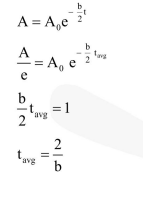# If a simple pendulum has Significant amplitude`
Question:

If a simple pendulum has Significant amplitude (up to a factor of $1 /$ e of original) only in the period between $t=0 \mathrm{~s}$ to $\mathrm{t}=\tau \mathrm{s}$, then $\tau$ may be called the average life ofthe pendulum. When the spherical bob of the pendulum suffers a retardation (due to viscous drag) proportional to its velocity, with " $b$ ' as the constant of proportionality, the average lifetime ofthe pendulum is (assuming damping is small) in seconds:

1. $\frac{1}{b}$

2. $\frac{2}{b}$

3. $\frac{0.693}{b}$

4. b

Correct Option: , 2

Solution: